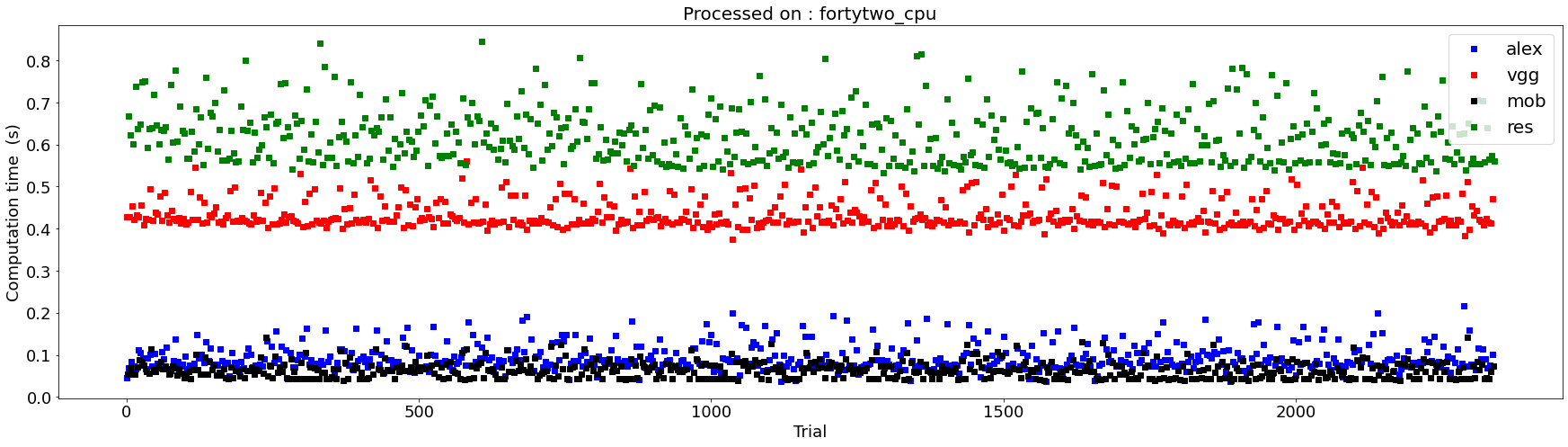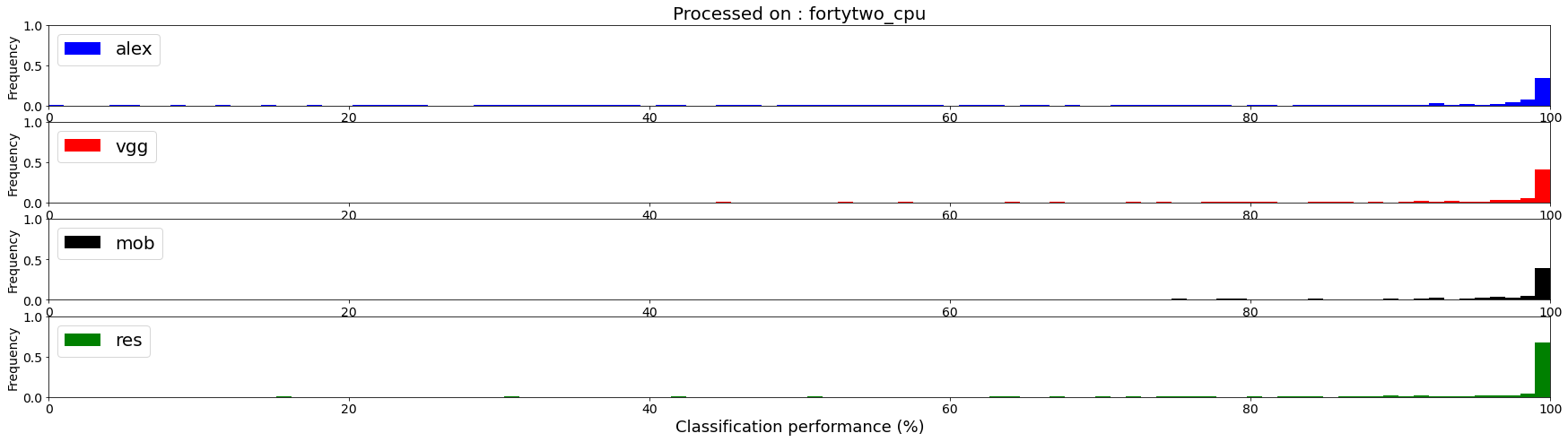# Benchmarking CNNs

Hi! I am Jean-Nicolas Jérémie and the goal of this benchmark is to offer a comparison between differents pre-trained image recognition's networks based on the Imagenet dataset wich allows to work on naturals images for \$1000\$ labels. These different networks tested here are taken from the `torchvision.models` library : `AlexNet`, `VGG16`, `MobileNetV2` and `ResNet101`.

Our use case is to measure the performance of a system which receives a sequence of images and has to make a decision as soon as possible, hence with `batch_size=1`. Specifically, we wish also to compare different computing architectures such as CPUs, desktop GPUs or other more exotic platform such as the Jetson TX2 (experiment 1). Additionally, we will implement some image transformations as up/down-sampling (experiment 2) or transforming to grayscale (experiment 3) to quantify their influence on the accuracy and computation time of each network.

In this notebook, I will use the Pytorch library for running the networks and the pandas library to collect and display the results. This notebook was done during a master 1 internship at the Neurosciences Institute of Timone (INT) under the supervision of Laurent PERRINET. It is curated in the following github repo.

## Initialization of the benchmark¶

Our coding strategy is to build up a small libray as a package of scripts in the `DCNN_benchmark` folder and to run all calls to that library from this notebook. This organization will be useful to run on specific hardware such as the Jetson card on the one hand and to visualize results in the notebook, on the other hand.

In :
```%matplotlib inline
%mkdir -p DCNN_benchmark
```

### Importing libraries; definition of the dataset¶

In :
```%%writefile DCNN_benchmark/init.py

# Importing libraries
import os
import time
from time import strftime,gmtime
import json
import time
import numpy as np
import imageio
from numpy import random
from torchvision.datasets import ImageFolder
# to plot
import matplotlib.pyplot as plt
# to store results
import pandas as pd

# figure's variables
fig_width = 20
phi = (np.sqrt(5)+1)/2 # golden ratio
phi = phi**2
colors = ['b', 'r', 'k','g']

# host & date's variables
# HOST = os.uname()
HOST = 'jnjer-HP-Pavilion-Notebook'
HOST = 'fortytwo'
#datetag = strftime("%Y-%m-%d", gmtime())
datetag = '2020-08-27'

#dataset configuration

image_size = 256 # default image resolution
image_sizes = 2**np.arange(6, 10) # resolutions explored in experiment 2

N_images_per_class = 10
#i_labels = random.randint(1000, size=(N_labels)) # Random choice
i_labels = [409, 530, 892, 487, 920, 704, 879, 963, 646, 620 ] # Pre-selected classes
N_labels = len(i_labels)

id_dl = ''
root = 'data'
folder = 'imagenet_classes_100'
path = os.path.join(root, folder) # data path

labels = [line.strip() for line in f.readlines()]
labels.split(', ')
labels = [label.split(', ').lower().replace('_', ' ') for label in labels]

# a reverse look-up-table giving the index of a given label (within the whole set of imagenet labels)
reverse_labels = {}
for i_label, label in enumerate(labels):
reverse_labels[label] = i_label
# a reverse look-up-table giving the index of a given i_label (within the sub-set of classes)
reverse_i_labels = {}
for i_label, label in enumerate(i_labels):
reverse_i_labels[label] = i_label

def pprint(message):
print('-'*len(message))
print(message)
print('-'*len(message))

pprint('List of Pre-selected classes')
# choosing the selected classes for recognition
for i_label in i_labels:
print('label', i_label, '=', labels[i_label])
for key in name:
if name[key]['class_name'] == labels[i_label]:
id_dl += key + ' '
pprint('label IDs = ' + str(id_dl) )
```
```Overwriting DCNN_benchmark/init.py
```
In :
```%run DCNN_benchmark/init.py
```
```----------------------------
List of Pre-selected classes
----------------------------
label 409 = analog clock
label 530 = digital clock
label 892 = wall clock
label 487 = cellular telephone
label 920 = traffic light
label 704 = parking meter
label 879 = umbrella
label 963 = pizza
label 646 = maze
label 620 = laptop
----------------------------------------------------------------------------------------------------------------
label IDs = n02708093 n03196217 n04548280 n02992529 n06874185 n03891332 n04507155 n07873807 n03733281 n03642806
----------------------------------------------------------------------------------------------------------------
```
In :
```if HOST == 'fortytwo':
do_local = False
python_exec = "KMP_DUPLICATE_LIB_OK=TRUE python3"
else :
do_local =True
```

We use an ImageNet dataloader to populate a dataset based on the pre-selected or randoms classes listed in the `DCNN_benchmark/init.py` file.

In :
```scriptname = 'DCNN_benchmark/dataset.py'
```
In :
```%%writefile {scriptname}

from DCNN_benchmark.init import *

# check if the folder exist
if os.path.isdir(path):
list_dir = os.listdir(path)
print("The folder " , folder, " already exists, it includes: ", list_dir)

# no folder, creating one
else :
print(f"No existing path match for this folder, creating a folder at {path}")
os.makedirs(path)

if len(list_dir) < N_labels :
print('Command to run : ', cmd)
os.system(cmd) # running it
list_dir = os.listdir(path)

elif len(os.listdir(path)) == N_labels :
print(f'The folder already contains : {len(list_dir)} classes')

else : # if there are to many folders delete some
print('The folder have to many classes, deleting some')
for elem in os.listdir(path):
contenu = os.listdir(f'{path}/{elem}')
if len(os.listdir(path)) > N_labels :
for x in contenu:
os.remove(f'{path}/{elem}/{x}') # delete exces folders
try:
os.rmdir(f'{path}/{elem}')
except:
os.remove(f'{path}/{elem}')
list_dir = os.listdir(path)
print("Now the folder " , folder, f" contains :", os.listdir(path))
```
```Overwriting DCNN_benchmark/dataset.py
```
In :
```if do_local:
%run {scriptname}
else:
!python3 {scriptname}
```
```----------------------------
List of Pre-selected classes
----------------------------
label 409 = analog clock
label 530 = digital clock
label 892 = wall clock
label 487 = cellular telephone
label 920 = traffic light
label 704 = parking meter
label 879 = umbrella
label 963 = pizza
label 646 = maze
label 620 = laptop
----------------------------------------------------------------------------------------------------------------
label IDs = n02708093 n03196217 n04548280 n02992529 n06874185 n03891332 n04507155 n07873807 n03733281 n03642806
----------------------------------------------------------------------------------------------------------------
The folder  imagenet_classes_100  already exists, it includes:  ['digital clock', 'analog clock', 'wall clock', 'parking meter', 'cellular telephone', 'maze', 'umbrella', 'laptop', 'pizza', 'traffic light']
The folder already contains : 10 classes
```

### Pre-trained network's import¶

Here we worked on four differents pre-trained networks `Alexnet`, `Mobilenet`, `Resnet101` and `VGG16`:

In :
```scriptname = 'DCNN_benchmark/models.py'
```
In :
```%%writefile {scriptname}

from DCNN_benchmark.init import *

import torch
import torchvision
import torchvision.transforms as transforms

# transform function for input's image processing
transform = transforms.Compose([
transforms.Resize(int(image_size)),      # Resize the image to image_size x image_size pixels size.
transforms.CenterCrop(int(image_size-20)),  # Crop the image to (image_size-20) x (image_size-20) pixels around the center.
transforms.ToTensor(),       # Convert the image to PyTorch Tensor data type.
transforms.Normalize(        # Normalize the image by adjusting
mean=[0.485, 0.456, 0.406],  #  its average and
std=[0.229, 0.224, 0.225]    #  its standard deviation at the specified values.
)])

image_dataset = ImageFolder(path, transform=transform) # save the dataset

# imports networks with weights
models = {} # get model's names

models['alex'] = torchvision.models.alexnet(pretrained=True)
models['vgg'] = torchvision.models.vgg16(pretrained=True)
models['mob'] = torchvision.models.mobilenet_v2(pretrained=True)
models['res'] = torchvision.models.resnext101_32x8d(pretrained=True)

# Select a device (CPU or CUDA)
device = torch.device("cuda:0" if torch.cuda.is_available() else "cpu")
for name in models.keys():
models[name].to(device)
```
```Overwriting DCNN_benchmark/models.py
```
In :
```%run {scriptname}
```
```----------------------------
List of Pre-selected classes
----------------------------
label 409 = analog clock
label 530 = digital clock
label 892 = wall clock
label 487 = cellular telephone
label 920 = traffic light
label 704 = parking meter
label 879 = umbrella
label 963 = pizza
label 646 = maze
label 620 = laptop
----------------------------------------------------------------------------------------------------------------
label IDs = n02708093 n03196217 n04548280 n02992529 n06874185 n03891332 n04507155 n07873807 n03733281 n03642806
----------------------------------------------------------------------------------------------------------------
```

## Experiment 1: Image processing and recognition for differents labels :¶

To recover the classification confidence of the models according to the classes on which they have been trained, i.e. the \$1000\$ classes of the `ImageNet` library, the `softmax` mathematical function is added at the last layer of the networks. The `softmax` function is a function which takes a vector of real values (here represented by a 1-D tensor) of dimension `K` (here `K=1000` trained classes) and returns for each of these values a normalized propability between \$0\$ and \$1\$ with a sum equal to \$1\$. Thus, all the classes are represented in the final vector and a low probability would then be a proof of absence for instance. A careful reading of the original imagenet paper shows that this probability reflects the response of users to questions such as "Is there a Burmese cat in the images?" when presented an image (retrieved on internet) which is likely to include "Burmese cat".

Here, we are interested in a sub-set of such classes. Nevertheless, the recognition being carried out on so-called "natural" images of the irrelevant classes could "mask" the recognition of those of interest. To reduce this effect, we have applied a slight modification to the output `softmax` function, by assuming that we know a priori that the image belongs to one (and only one) category from the sub-set, but that we do not know which one. As a consequence, it does not recover a vector of \$K = 1000\$ but of \$K = N_{labels}\$. As a consequence, the probabilities obtained would correspond to a confidence of classification discriminating only the classes of interest and can be compared to a chance level of \$1 / N_{labels}\$.

For further statistical analyses, we extract these differents factors (like the accuracy and the processing time for differents datasets at differents resolution) in a `pandas` object.

In :
```scriptname = 'experiment_basic.py'
```
In :
```%%writefile {scriptname}

#import model's script and set the output file
from DCNN_benchmark.models import *
filename = f'results/{datetag}_results_1_{HOST}.json'

try:
except:
df = pd.DataFrame([], columns=['model', 'perf', 'fps', 'time', 'label', 'i_label', 'i_image', 'filename', 'device'])
i_trial = 0

# image preprocessing
for i_image, (data, label) in enumerate(image_dataset):
for name in models.keys():
model = models[name]
model.eval()
tic = time.time()
out = model(data.unsqueeze(0).to(device)).squeeze(0)
percentage = torch.nn.functional.softmax(out[i_labels], dim=0) * 100
_, indices = torch.sort(percentage, descending=True)
dt = time.time() - tic
i_label_top = reverse_labels[image_dataset.classes[label]]
perf_ = percentage[reverse_i_labels[i_label_top]].item()
df.loc[i_trial] = {'model':name, 'perf':perf_, 'time':dt, 'fps': 1/dt,
'label':labels[i_label_top], 'i_label':i_label_top,
'i_image':i_image, 'filename':image_dataset.imgs[i_image], 'device':str(device)}
print(f'The {name} model get {labels[i_label_top]} at {perf_:.2f} % confidence in {dt:.3f} seconds')
i_trial += 1
df.to_json(filename)
```
```Overwriting experiment_basic.py
```
In :
```if do_local:
%run {scriptname}
else:
!{python_exec} {scriptname}
```
```----------------------------
List of Pre-selected classes
----------------------------
label 409 = analog clock
label 530 = digital clock
label 892 = wall clock
label 487 = cellular telephone
label 920 = traffic light
label 704 = parking meter
label 879 = umbrella
label 963 = pizza
label 646 = maze
label 620 = laptop
----------------------------------------------------------------------------------------------------------------
label IDs = n02708093 n03196217 n04548280 n02992529 n06874185 n03891332 n04507155 n07873807 n03733281 n03642806
----------------------------------------------------------------------------------------------------------------
```

### Image recognition on differents labels display :¶

Here we collect our results, we can already display all the data in a table

In :
```filename = f'results/{datetag}_results_1_{HOST}.json'
df
```
Out:
model perf fps time label i_label i_image filename device
0 alex 30.283058 22.265360 0.044913 analog clock 409 0 data/imagenet_classes_100/analog clock/1021913... cpu
1 vgg 62.287682 2.338869 0.427557 analog clock 409 0 data/imagenet_classes_100/analog clock/1021913... cpu
2 mob 42.539902 18.122563 0.055180 analog clock 409 0 data/imagenet_classes_100/analog clock/1021913... cpu
3 res 70.461670 1.496365 0.668286 analog clock 409 0 data/imagenet_classes_100/analog clock/1021913... cpu
4 alex 7.313114 14.566287 0.068652 analog clock 409 1 data/imagenet_classes_100/analog clock/1036540... cpu
... ... ... ... ... ... ... ... ... ...
2335 res 81.857353 1.745690 0.572839 wall clock 892 583 data/imagenet_classes_100/wall clock/944534279... cpu
2336 alex 76.701584 9.910013 0.100908 wall clock 892 584 data/imagenet_classes_100/wall clock/97880360_... cpu
2337 vgg 78.698418 2.124301 0.470743 wall clock 892 584 data/imagenet_classes_100/wall clock/97880360_... cpu
2338 mob 46.693691 13.741139 0.072774 wall clock 892 584 data/imagenet_classes_100/wall clock/97880360_... cpu
2339 res 83.952156 1.785157 0.560175 wall clock 892 584 data/imagenet_classes_100/wall clock/97880360_... cpu

2340 rows × 9 columns

A display of the differents computation time of each models on the same dataset for the sequence of trials :

In :
```fig, axs = plt.subplots(figsize=(30, fig_width/phi))
plt.xticks(fontsize=18)
plt.yticks(fontsize=18)

for color, name in zip(colors, models.keys()):
axs.set_ylabel('Computation time  (s)', size= 18)
axs.set_xlabel('Trial', size= 18)
#axs.set_ylim(0, 1)
df[df['model']==name]['time'].plot(label=name, color=color, marker='s', lw=0)
axs.legend(loc=0, fontsize = 20)
axs.set_title('Processed on : '  + HOST + '_' + str(df['device']), size = 20)
```This graph shows the frequency of the classification performance for our four models.

In :
```fig, axs = plt.subplots(len(models), 1, figsize=(30, fig_width/phi))
plt.xticks(fontsize=18)
plt.yticks(fontsize=18)
for ax, color, name in zip(axs, colors, models.keys()):
ax.set_ylabel('Frequency', fontsize=14)
df[df['model']==name]['perf'].plot.hist(bins=np.linspace(0, 100, 100), lw=1, label=name,ax=ax, color=color, density=True)
ax.legend(loc='upper left', fontsize = 20)
ax.set_xlim(0, 100)
ax.set_ylim(0, 1)
ax.tick_params(axis='x', labelsize=14)
ax.tick_params(axis='y', labelsize=14)
axs[-1].set_xlabel('Classification performance (%)', size= 18)
axs.set_title('Processed on : ' + HOST + '_' + str(df['device']), size = 20);
```Here we display the 64 worsts classification performance, all model combined :

In :
```N_image_i = 8
N_image_j = 8
fig, axs = plt.subplots(N_image_i, N_image_j, figsize=(21, 21))
for i, idx in enumerate(df["perf"].argsort()[:(N_image_i*N_image_j)]):
ax = axs[i%N_image_i][i//N_image_i]